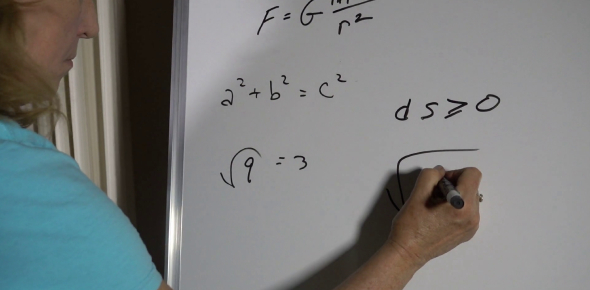# Writing A System Of Equations

5 QuestionsSettingsWrite a system of linear equations for each of the word problems given.

Related Topics
• 1.
Write the linear system of equations for the story problem below: Find the value of two numbers if their sum is 12 and their difference is 4.
• A.
• B.
• C.
• D.
• 2.
Write the linear system of equations for the story problem below: The school that Stefan goes to is selling tickets to a choral performance. On the first day of ticket sales the school sold 3 senior citizen tickets and 1 child ticket for a total of \$38. The school took in \$52 on the second day by selling 3 senior citizen tickets and 2 child tickets. Find the price of a senior citizen ticket and the price of a child ticket.
• A.
• B.
• C.
• 3.
Write the linear system of equations for the story problem below: The state fair is a popular field trip destination. This year the senior class at High School A and the senior class at High School B both planned trips there. The senior class at High School A rented and filled 8 vans and 8 buses with 240 students. High School B rented and filled 4 vans and 1 bus with 54 students. Every van had the same number of students in it as did the buses.
• A.
• B.
• C.
• 4.
Write the linear system of equations for the story problem below: Matt and Ming are selling fruit for a school fundraiser. Customers can buy small boxes of oranges and large boxes of oranges. Matt sold 3 small boxes of oranges and 14 large boxes of oranges for a total of \$203. Ming sold 11 small boxes of oranges and 11 large boxes of oranges for a total of \$220. Find the cost each of one small box of oranges and one large box of oranges.
• A.
• B.
• C.
• 5.
Write the linear system of equations for the story problem below: The difference of two numbers is 3. Their sum is 13.
• A.
• B.
• C.